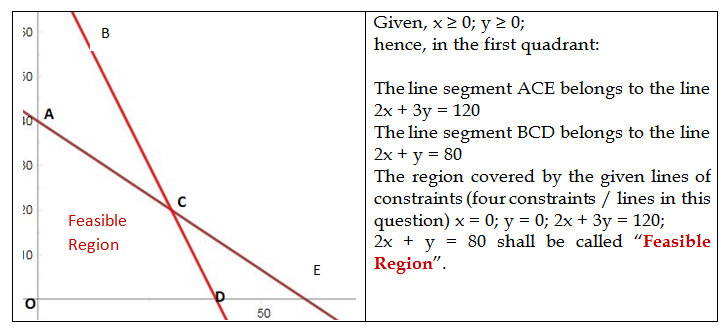top of page

# What is Optimal Feasible Region in solving LPP, and how to solve an LPP having feasible region in th

In my first three blogs on Linear Programming, I gave you an idea of Linear Programming Problems (LPP), of some basic terms of LPP and of the terms to be used in (graphical) technique of solving an LPP. In this blog, the final lesson on the understanding of solving LPP shall be taken up and then we shall do questions.

In the previous blog, you understood the feasible region and feasible solutions. Of course, there are an infinite number of feasible solutions available in the feasible region and we have to get to the one which gives us optimized solution (that is, the objective function being either most positive or most negative in terms of values of x and y, the decision variables.

I shall begin with giving you a theorem (without proof) of utmost importance in solving LPP with graphical method.

Theorem 1: Let R be the feasible region (convex polygon) for a linear programming Problem and let Z = ax + by be the objective function. When Z has an optimal value (maximum or minimum), where the variables x and y are subject to constraints described by linear inequalities, this optimal value must occur at a corner point (vertex) of the feasible region.

Implication of Theorem 1

In our previous blog, for the question:

A company manufactures two types of screws: type A and type B. All the screws have to pass through a threading machine and a slotting machine. The box of type A screws requires 4 minutes on the threading machine and 6 minutes on this slotting machine. A box of type B screws requires 6 minutes on the threading machine and 3 minutes on this slotting machine. In a week, each machine is available for 4 hours on any day. On selling these screws, the company gets a profit of Rs. 7 per box on type A screws and Rs. 10 per box on type B screws. Formulate this problem as a LPP to maximize the profit to the company.

The LPP was: Max z = 7x + 10y

x ≥ 0; y ≥ 0; 2x + 3y ≤ 120; 2x + y ≤ 80; and had found that the coordinates of the corner points of the feasible region formed by the intersection of straight lines, x = 0, y = 0, 2x + 3y = 120 were: O (0,0); A (0,40); C (30,20); D (40,0)

This was explained in previous blog giving following graph:Thus the optimal feasible solution will be either (0,0) or (0,40) or (30,20) or(40,0).

Now, we shall construct a table for finding out the value of z for values of x and y for these four corner points.The optimum function is maximum at x = 30 and y = 20. And maximum profit at these decision variable is z = 410. Answer.

Thus for a feasible region having shape of a convex polygon for a linear programming Problem, we have a flowchart for solving the LPP:

1. We shall find the feasible region of the LPP by converting the inequalities in linear equations and determine the corner points (i.e. vertices). This can be done either by simple inspection of the equations of the straight lines or by solving them.

2. For the values of x and y (the decision variables), we shall work out the value of the objective function z = ax + by.

But what about the case when the feasible region is not a convex polygon? We shall consider it in the next blog on this series of Linear Programming.

Tags:

###### Featured Posts
Check back soon
Once posts are published, you’ll see them here.